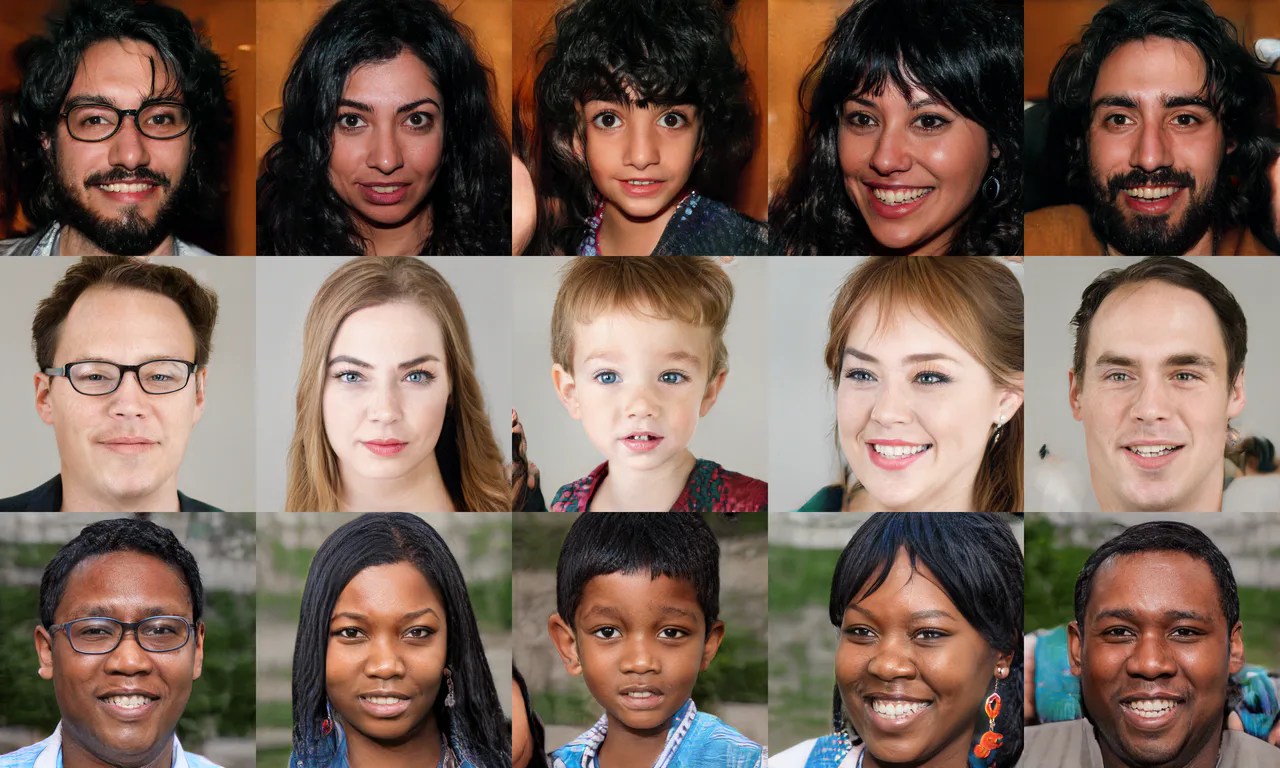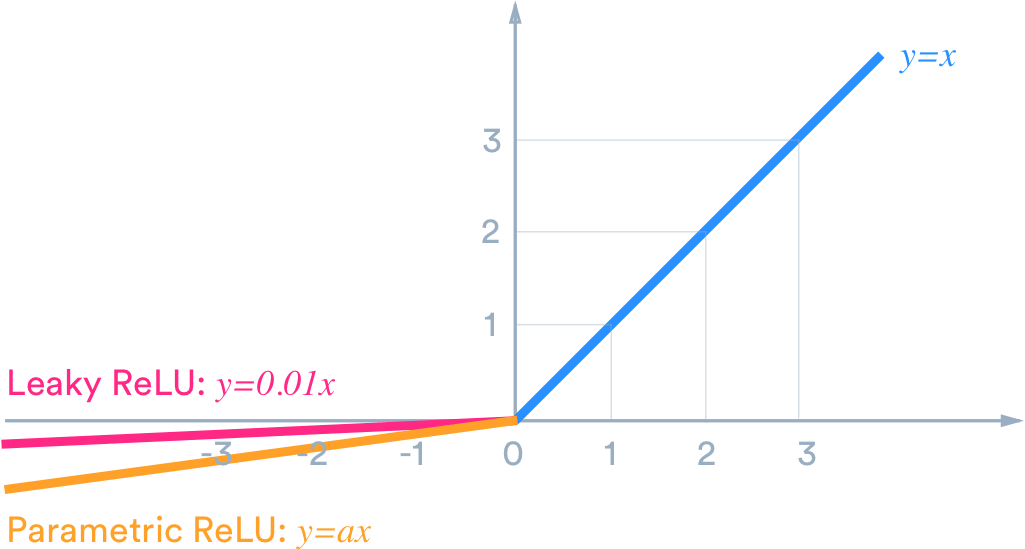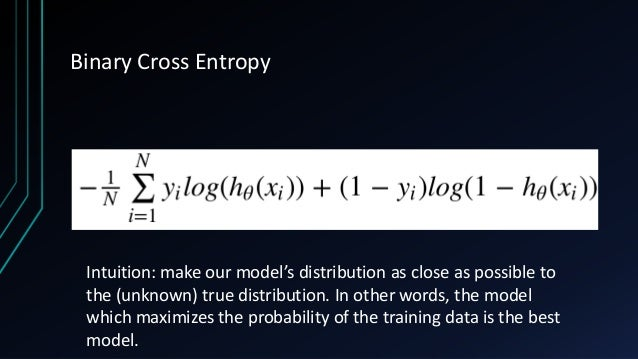Learn data science and machine learning by building real-world projects on Jovian

Training Generative Adversarial Networks (GANs) in PyTorch

Part 7 of "Deep Learning with Pytorch: Zero to GANs"

This tutorial series is a hands-on beginner-friendly introduction to deep learning using PyTorch, an open-source neural networks library. These tutorials take a practical and coding-focused approach. The best way to learn the material is to execute the code and experiment with it yourself. Check out the full series here:

How to run the code

This tutorial is an executable Jupyter notebook hosted on Jovian. You can run this tutorial and experiment with the code examples in a couple of ways: using free online resources (recommended) or on your computer.

Option 1: Running using free online resources (1-click, recommended)

The easiest way to start executing the code is to click the Run button at the top of this page and select Run on Colab. Google Colab is a free online platform for running Jupyter notebooks using Google's cloud infrastructure. You can also select "Run on Binder" or "Run on Kaggle" if you face issues running the notebook on Google Colab.

Option 2: Running on your computer locally

To run the code on your computer locally, you'll need to set up Python, download the notebook and install the required libraries. We recommend using the Conda distribution of Python. Click the Run button at the top of this page, select the Run Locally option, and follow the instructions.

Using a GPU for faster training

You can use a Graphics Processing Unit (GPU) to train your models faster if your execution platform is connected to a GPU manufactured by NVIDIA. Follow these instructions to use a GPU on the platform of your choice:

• Google Colab: Use the menu option "Runtime > Change Runtime Type" and select "GPU" from the "Hardware Accelerator" dropdown.
• Kaggle: In the "Settings" section of the sidebar, select "GPU" from the "Accelerator" dropdown. Use the button on the top-right to open the sidebar.
• Binder: Notebooks running on Binder cannot use a GPU, as the machines powering Binder aren't connected to any GPUs.
• Linux: If your laptop/desktop has an NVIDIA GPU (graphics card), make sure you have installed the NVIDIA CUDA drivers.
• Windows: If your laptop/desktop has an NVIDIA GPU (graphics card), make sure you have installed the NVIDIA CUDA drivers.
• macOS: macOS is not compatible with NVIDIA GPUs

If you do not have access to a GPU or aren't sure what it is, don't worry, you can execute all the code in this tutorial just fine without a GPU.

Introduction to Generative Modeling

Deep neural networks are used mainly for supervised learning: classification or regression. Generative Adversarial Networks or GANs, however, use neural networks for a very different purpose: Generative modeling

Generative modeling is an unsupervised learning task in machine learning that involves automatically discovering and learning the regularities or patterns in input data in such a way that the model can be used to generate or output new examples that plausibly could have been drawn from the original dataset. - Source

To get a sense of the power of generative models, just visit thispersondoesnotexist.com. Every time you reload the page, a new image of a person's face is generated on the fly. The results are pretty fascinating:While there are many approaches used for generative modeling, a Generative Adversarial Network takes the following approach:There are two neural networks: a Generator and a Discriminator. The generator generates a "fake" sample given a random vector/matrix, and the discriminator attempts to detect whether a given sample is "real" (picked from the training data) or "fake" (generated by the generator). Training happens in tandem: we train the discriminator for a few epochs, then train the generator for a few epochs, and repeat. This way both the generator and the discriminator get better at doing their jobs.

GANs however, can be notoriously difficult to train, and are extremely sensitive to hyperparameters, activation functions and regularization. In this tutorial, we'll train a GAN to generate images of anime characters' faces.We'll use the Anime Face Dataset, which consists of over 63,000 cropped anime faces. Note that generative modeling is an unsupervised learning task, so the images do not have any labels. Most of the code in this tutorial is based on this notebook.

project_name = '06b-anime-dcgan'
# Uncomment and run the appropriate command for your operating system, if required
# No installation is reqiured on Google Colab / Kaggle notebooks

# Linux / Binder / Windows (No GPU)

# Linux / Windows (GPU)

# MacOS (NO GPU)
# !pip install numpy matplotlib torch torchvision torchaudio

2. Scroll down to the "API" section and click "Create New API Token". This will download a file kaggle.json with the following contents:

Note that you need to download the kaggle.json file only once. On Google Colab, you can also upload the kaggle.json file using the files tab, and the credentials will be read automatically.

import opendatasets as od

dataset_url = 'https://www.kaggle.com/splcher/animefacedataset'
100%|██████████| 395M/395M [00:05<00:00, 79.4MB/s]

The dataset has a single folder called images which contains all 63,000+ images in JPG format.

import os

DATA_DIR = './animefacedataset'
print(os.listdir(DATA_DIR))
['images']
print(os.listdir(DATA_DIR+'/images')[:10])
['16490_2006.jpg', '60428_2018.jpg', '39263_2012.jpg', '12752_2005.jpg', '19303_2007.jpg', '16175_2006.jpg', '48709_2014.jpg', '15538_2006.jpg', '28010_2009.jpg', '17006_2007.jpg']

Let's load this dataset using the ImageFolder class from torchvision. We will also resize and crop the images to 64x64 px, and normalize the pixel values with a mean & standard deviation of 0.5 for each channel. This will ensure that pixel values are in the range (-1, 1), which is more convenient for training the discriminator. We will also create a data loader to load the data in batches.

from torchvision.datasets import ImageFolder
import torchvision.transforms as T
image_size = 64
batch_size = 128
stats = (0.5, 0.5, 0.5), (0.5, 0.5, 0.5)
train_ds = ImageFolder(DATA_DIR, transform=T.Compose([
T.Resize(image_size),
T.CenterCrop(image_size),
T.ToTensor(),
T.Normalize(*stats)]))

train_dl = DataLoader(train_ds, batch_size, shuffle=True, num_workers=3, pin_memory=True)
/usr/local/lib/python3.7/dist-packages/torch/utils/data/dataloader.py:481: UserWarning: This DataLoader will create 3 worker processes in total. Our suggested max number of worker in current system is 2, which is smaller than what this DataLoader is going to create. Please be aware that excessive worker creation might get DataLoader running slow or even freeze, lower the worker number to avoid potential slowness/freeze if necessary. cpuset_checked))

Let's create helper functions to denormalize the image tensors and display some sample images from a training batch.

import torch
from torchvision.utils import make_grid
import matplotlib.pyplot as plt
%matplotlib inline
def denorm(img_tensors):
return img_tensors * stats + stats
def show_images(images, nmax=64):
fig, ax = plt.subplots(figsize=(8, 8))
ax.set_xticks([]); ax.set_yticks([])
ax.imshow(make_grid(denorm(images.detach()[:nmax]), nrow=8).permute(1, 2, 0))

def show_batch(dl, nmax=64):
for images, _ in dl:
show_images(images, nmax)
break
show_batch(train_dl)
/usr/local/lib/python3.7/dist-packages/torch/utils/data/dataloader.py:481: UserWarning: This DataLoader will create 3 worker processes in total. Our suggested max number of worker in current system is 2, which is smaller than what this DataLoader is going to create. Please be aware that excessive worker creation might get DataLoader running slow or even freeze, lower the worker number to avoid potential slowness/freeze if necessary. cpuset_checked))import jovian
jovian.commit(project=project_name, environment=None)
[jovian] Detected Colab notebook... [jovian] Please enter your API key ( from https://jovian.ai/ ): API KEY:

Using a GPU

To seamlessly use a GPU, if one is available, we define a couple of helper functions (get_default_device & to_device) and a helper class DeviceDataLoader to move our model & data to the GPU, if one is available.

def get_default_device():
"""Pick GPU if available, else CPU"""
if torch.cuda.is_available():
else:

def to_device(data, device):
"""Move tensor(s) to chosen device"""
if isinstance(data, (list,tuple)):
return [to_device(x, device) for x in data]
return data.to(device, non_blocking=True)

"""Wrap a dataloader to move data to a device"""
def __init__(self, dl, device):
self.dl = dl
self.device = device

def __iter__(self):
"""Yield a batch of data after moving it to device"""
for b in self.dl:
yield to_device(b, self.device)

def __len__(self):
"""Number of batches"""
return len(self.dl)

Based on where you're running this notebook, your default device could be a CPU (torch.device('cpu')) or a GPU (torch.device('cuda')).

device = get_default_device()
device

We can now move our training data loader using DeviceDataLoader for automatically transferring batches of data to the GPU (if available).

Discriminator Network

The discriminator takes an image as input, and tries to classify it as "real" or "generated". In this sense, it's like any other neural network. We'll use a convolutional neural networks (CNN) which outputs a single number output for every image. We'll use stride of 2 to progressively reduce the size of the output feature map.import torch.nn as nn
discriminator = nn.Sequential(
# in: 3 x 64 x 64

nn.Conv2d(3, 64, kernel_size=4, stride=2, padding=1, bias=False),
nn.BatchNorm2d(64),
nn.LeakyReLU(0.2, inplace=True),
# out: 64 x 32 x 32

nn.Conv2d(64, 128, kernel_size=4, stride=2, padding=1, bias=False),
nn.BatchNorm2d(128),
nn.LeakyReLU(0.2, inplace=True),
# out: 128 x 16 x 16

nn.Conv2d(128, 256, kernel_size=4, stride=2, padding=1, bias=False),
nn.BatchNorm2d(256),
nn.LeakyReLU(0.2, inplace=True),
# out: 256 x 8 x 8

nn.Conv2d(256, 512, kernel_size=4, stride=2, padding=1, bias=False),
nn.BatchNorm2d(512),
nn.LeakyReLU(0.2, inplace=True),
# out: 512 x 4 x 4

nn.Conv2d(512, 1, kernel_size=4, stride=1, padding=0, bias=False),
# out: 1 x 1 x 1

nn.Flatten(),
nn.Sigmoid())

Note that we're using the Leaky ReLU activation for the discriminator.Different from the regular ReLU function, Leaky ReLU allows the pass of a small gradient signal for negative values. As a result, it makes the gradients from the discriminator flows stronger into the generator. Instead of passing a gradient (slope) of 0 in the back-prop pass, it passes a small negative gradient. - Source

Just like any other binary classification model, the output of the discriminator is a single number between 0 and 1, which can be interpreted as the probability of the input image being real i.e. picked from the original dataset.

Let's move the discriminator model to the chosen device.

discriminator = to_device(discriminator, device)

Generator Network

The input to the generator is typically a vector or a matrix of random numbers (referred to as a latent tensor) which is used as a seed for generating an image. The generator will convert a latent tensor of shape (128, 1, 1) into an image tensor of shape 3 x 28 x 28. To achive this, we'll use the ConvTranspose2d layer from PyTorch, which is performs to as a transposed convolution (also referred to as a deconvolution). Learn morelatent_size = 128
generator = nn.Sequential(
# in: latent_size x 1 x 1

nn.ConvTranspose2d(latent_size, 512, kernel_size=4, stride=1, padding=0, bias=False),
nn.BatchNorm2d(512),
nn.ReLU(True),
# out: 512 x 4 x 4

nn.ConvTranspose2d(512, 256, kernel_size=4, stride=2, padding=1, bias=False),
nn.BatchNorm2d(256),
nn.ReLU(True),
# out: 256 x 8 x 8

nn.ConvTranspose2d(256, 128, kernel_size=4, stride=2, padding=1, bias=False),
nn.BatchNorm2d(128),
nn.ReLU(True),
# out: 128 x 16 x 16

nn.ConvTranspose2d(128, 64, kernel_size=4, stride=2, padding=1, bias=False),
nn.BatchNorm2d(64),
nn.ReLU(True),
# out: 64 x 32 x 32

nn.ConvTranspose2d(64, 3, kernel_size=4, stride=2, padding=1, bias=False),
nn.Tanh()
# out: 3 x 64 x 64
)

We use the TanH activation function for the output layer of the generator."The ReLU activation (Nair & Hinton, 2010) is used in the generator with the exception of the output layer which uses the Tanh function. We observed that using a bounded activation allowed the model to learn more quickly to saturate and cover the color space of the training distribution. Within the discriminator we found the leaky rectified activation (Maas et al., 2013) (Xu et al., 2015) to work well, especially for higher resolution modeling." - Source

Note that since the outputs of the TanH activation lie in the range [-1,1], we have applied the similar transformation to the images in the training dataset. Let's generate some outputs using the generator and view them as images by transforming and denormalizing the output.

xb = torch.randn(batch_size, latent_size, 1, 1) # random latent tensors
fake_images = generator(xb)
print(fake_images.shape)
show_images(fake_images)

As one might expect, the output from the generator is basically random noise, since we haven't trained it yet.

Let's move the generator to the chosen device.

generator = to_device(generator, device)

Discriminator Training

Since the discriminator is a binary classification model, we can use the binary cross entropy loss function to quantify how well it is able to differentiate between real and generated images.def train_discriminator(real_images, opt_d):

# Pass real images through discriminator
real_preds = discriminator(real_images)
real_targets = torch.ones(real_images.size(0), 1, device=device)
real_loss = F.binary_cross_entropy(real_preds, real_targets)
real_score = torch.mean(real_preds).item()

# Generate fake images
latent = torch.randn(batch_size, latent_size, 1, 1, device=device)
fake_images = generator(latent)

# Pass fake images through discriminator
fake_targets = torch.zeros(fake_images.size(0), 1, device=device)
fake_preds = discriminator(fake_images)
fake_loss = F.binary_cross_entropy(fake_preds, fake_targets)
fake_score = torch.mean(fake_preds).item()

# Update discriminator weights
loss = real_loss + fake_loss
loss.backward()
opt_d.step()
return loss.item(), real_score, fake_score

Here are the steps involved in training the discriminator.

• We expect the discriminator to output 1 if the image was picked from the real MNIST dataset, and 0 if it was generated using the generator network.

• We first pass a batch of real images, and compute the loss, setting the target labels to 1.

• Then we pass a batch of fake images (generated using the generator) pass them into the discriminator, and compute the loss, setting the target labels to 0.

• Finally we add the two losses and use the overall loss to perform gradient descent to adjust the weights of the discriminator.

It's important to note that we don't change the weights of the generator model while training the discriminator (opt_d only affects the discriminator.parameters())

Generator Training

Since the outputs of the generator are images, it's not obvious how we can train the generator. This is where we employ a rather elegant trick, which is to use the discriminator as a part of the loss function. Here's how it works:

• We generate a batch of images using the generator, pass the into the discriminator.

• We calculate the loss by setting the target labels to 1 i.e. real. We do this because the generator's objective is to "fool" the discriminator.

• We use the loss to perform gradient descent i.e. change the weights of the generator, so it gets better at generating real-like images to "fool" the discriminator.

Here's what this looks like in code.

def train_generator(opt_g):

# Generate fake images
latent = torch.randn(batch_size, latent_size, 1, 1, device=device)
fake_images = generator(latent)

# Try to fool the discriminator
preds = discriminator(fake_images)
targets = torch.ones(batch_size, 1, device=device)
loss = F.binary_cross_entropy(preds, targets)

# Update generator weights
loss.backward()
opt_g.step()

return loss.item()

Let's create a directory where we can save intermediate outputs from the generator to visually inspect the progress of the model. We'll also create a helper function to export the generated images.

from torchvision.utils import save_image
sample_dir = 'generated'
os.makedirs(sample_dir, exist_ok=True)
def save_samples(index, latent_tensors, show=True):
fake_images = generator(latent_tensors)
fake_fname = 'generated-images-{0:0=4d}.png'.format(index)
save_image(denorm(fake_images), os.path.join(sample_dir, fake_fname), nrow=8)
print('Saving', fake_fname)
if show:
fig, ax = plt.subplots(figsize=(8, 8))
ax.set_xticks([]); ax.set_yticks([])
ax.imshow(make_grid(fake_images.cpu().detach(), nrow=8).permute(1, 2, 0))

We'll use a fixed set of input vectors to the generator to see how the individual generated images evolve over time as we train the model. Let's save one set of images before we start training our model.

fixed_latent = torch.randn(64, latent_size, 1, 1, device=device)
save_samples(0, fixed_latent)
jovian.commit(project=project_name, environment=None)

Full Training Loop

Let's define a fit function to train the discriminator and generator in tandem for each batch of training data. We'll use the Adam optimizer with some custom parameters (betas) that are known to work well for GANs. We will also save some sample generated images at regular intervals for inspection.from tqdm.notebook import tqdm
import torch.nn.functional as F
def fit(epochs, lr, start_idx=1):
torch.cuda.empty_cache()

# Losses & scores
losses_g = []
losses_d = []
real_scores = []
fake_scores = []

# Create optimizers
opt_d = torch.optim.Adam(discriminator.parameters(), lr=lr, betas=(0.5, 0.999))
opt_g = torch.optim.Adam(generator.parameters(), lr=lr, betas=(0.5, 0.999))

for epoch in range(epochs):
for real_images, _ in tqdm(train_dl):
# Train discriminator
loss_d, real_score, fake_score = train_discriminator(real_images, opt_d)
# Train generator
loss_g = train_generator(opt_g)

# Record losses & scores
losses_g.append(loss_g)
losses_d.append(loss_d)
real_scores.append(real_score)
fake_scores.append(fake_score)

# Log losses & scores (last batch)
print("Epoch [{}/{}], loss_g: {:.4f}, loss_d: {:.4f}, real_score: {:.4f}, fake_score: {:.4f}".format(
epoch+1, epochs, loss_g, loss_d, real_score, fake_score))

# Save generated images
save_samples(epoch+start_idx, fixed_latent, show=False)

return losses_g, losses_d, real_scores, fake_scores

We are now ready to train the model. Try different learning rates to see if you can maintain the fine balance between the training the generator and the discriminator.

lr = 0.0002
epochs = 25
jovian.reset()
jovian.log_hyperparams(lr=lr, epochs=epochs)
history = fit(epochs, lr)
losses_g, losses_d, real_scores, fake_scores = history
jovian.log_metrics(loss_g=losses_g[-1],
loss_d=losses_d[-1],
real_score=real_scores[-1],
fake_score=fake_scores[-1])

Now that we have trained the models, we can save checkpoints.

# Save the model checkpoints
torch.save(generator.state_dict(), 'G.pth')
torch.save(discriminator.state_dict(), 'D.pth')

Here's how the generated images look, after the 1st, 5th and 10th epochs of training.

from IPython.display import Image
Image('./generated/generated-images-0001.png')
Image('./generated/generated-images-0005.png')
Image('./generated/generated-images-0010.png')
Image('./generated/generated-images-0020.png')
Image('./generated/generated-images-0025.png')

We can visualize the training process by combining the sample images generated after each epoch into a video using OpenCV.

import cv2
import os

vid_fname = 'gans_training.avi'

files = [os.path.join(sample_dir, f) for f in os.listdir(sample_dir) if 'generated' in f]
files.sort()

out = cv2.VideoWriter(vid_fname,cv2.VideoWriter_fourcc(*'MP4V'), 1, (530,530))
out.release()

Here's what it looks like:We can also visualize how the loss changes over time. Visualizing losses is quite useful for debugging the training process. For GANs, we expect the generator's loss to reduce over time, without the discriminator's loss getting too high.

plt.plot(losses_d, '-')
plt.plot(losses_g, '-')
plt.xlabel('epoch')
plt.ylabel('loss')
plt.legend(['Discriminator', 'Generator'])
plt.title('Losses');
plt.plot(real_scores, '-')
plt.plot(fake_scores, '-')
plt.xlabel('epoch')
plt.ylabel('score')
plt.legend(['Real', 'Fake'])
plt.title('Scores');

Save and Commit

We can upload the full snapshot of this experiment to Jovian:

• Jupyter notebook
• Hyperparameters & metrics
• Models weights
• Training video
import jovian
jovian.commit(project=project_name,
outputs=['G.pth', 'D.pth', 'gans_training.avi'],
environment=None)